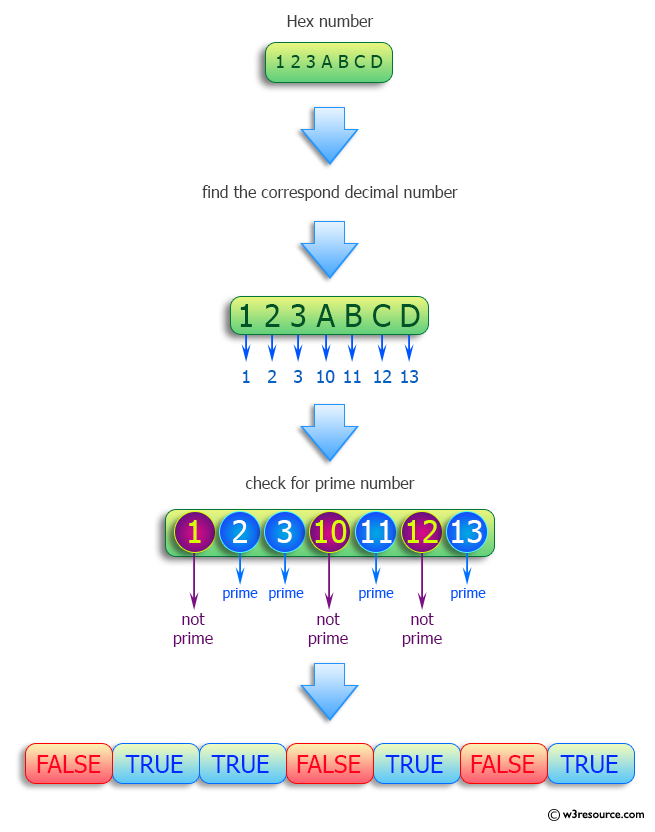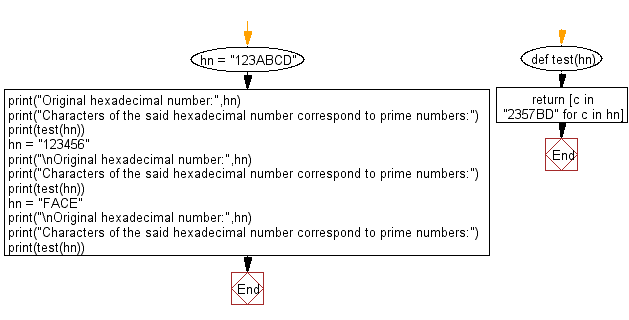﻿ Python: Determine which characters of a hexadecimal number correspond to prime numbers - w3resource# Python: Determine which characters of a hexadecimal number correspond to prime numbers

## Python Programming Puzzles: Exercise-44 with Solution

From Wikipedia:
The hexadecimal numeral system, often shortened to "hex", is a numeral system made up of 16 symbols (base 16). The standard numeral system is called decimal (base 10) and uses ten symbols: 0,1,2,3,4,5,6,7,8,9. Hexadecimal uses the decimal numbers and six extra symbols. There are no numerical symbols that represent values greater than nine, so letters taken from the English alphabet are used, specifically A, B, C, D, E and F. Hexadecimal A = decimal 10, and hexadecimal F = decimal 15.
A prime number (or a prime) is a natural number greater than 1 that is not a product of two smaller natural numbers. A natural number greater than 1 that is not prime is called a composite number. For example, 5 is prime because the only ways of writing it as a product, 1 × 5 or 5 × 1, involve 5 itself. However, 4 is composite because it is a product (2 × 2) in which both numbers are smaller than 4. Primes are central in number theory because of the fundamental theorem of arithmetic: every natural number greater than 1 is either a prime itself or can be factorized as a product of primes that is unique up to their order.
Write a Python program to find which characters of a hexadecimal number correspond to prime numbers.

```Input: 123ABCD
Output:
[False, True, True, False, True, False, True]

Input: 123456
Output:
[False, True, True, False, True, False]

Input: FACE
Output:
[False, False, False, False]
```

Pictorial Presentation:Sample Solution:

Python Code:

``````#License: https://bit.ly/3oLErEI

def test(hn):
return [c in "2357BD" for c in hn]
hn = "123ABCD"
print("Characters of the said hexadecimal number correspond to prime numbers:")
print(test(hn))
hn = "123456"
print("Characters of the said hexadecimal number correspond to prime numbers:")
print(test(hn))
hn = "FACE"
print("Characters of the said hexadecimal number correspond to prime numbers:")
print(test(hn))
``````

Sample Output:

```Original hexadecimal number: 123ABCD
Characters of the said hexadecimal number correspond to prime numbers:
[False, True, True, False, True, False, True]

Characters of the said hexadecimal number correspond to prime numbers:
[False, True, True, False, True, False]

Characters of the said hexadecimal number correspond to prime numbers:
[False, False, False, False]
```

Flowchart:## Visualize Python code execution:

The following tool visualize what the computer is doing step-by-step as it executes the said program:

Python Code Editor :

Have another way to solve this solution? Contribute your code (and comments) through Disqus.

What is the difficulty level of this exercise?

Test your Programming skills with w3resource's quiz.

﻿

## Python: Tips of the Day

Clamps num within the inclusive range specified by the boundary values x and y:

Example:

```def tips_clamp_num(num,x,y):
return max(min(num, max(x, y)), min(x, y))
print(tips_clamp_num(2, 4, 6))
print(tips_clamp_num(1, -1, -6))
```

Output:

```4
-1
```